# The Why’s and how’s of Machine Learning

The knowledge is the output of learning through the inseparable combination of theory and practice. It’s what remains in one’s experience from all the data which got shaped into what we call information. This process can be noticed throughout the different stages of our lives and it’s never limited to the academic journey.

What I’m aiming to express is that machine learning is nothing but a human logic tailored for more complex problems that surely require more computational capabilities.

“There are many different learning types and approaches to learning. To learn effectively it is important to tailor your study habits to your own needs and approach, this often means choosing techniques that work for you and evaluating them from time to time to determine if you need to try something new.” — The University Of British Columbia

The last quote represents the nature knowledge acquiring process which, as you may notice, is similar to CRISP-DM Methodology which I detailed in a previous article and which is essential to succeed in your data mining project.

___________________________________________________________________

### — — — — —What’s machine learning — — — — — — — -

To define Machine learning, its is a set of algorithms that are included in the many operations like the Data Mining process and which help you transform your raw data into knowledge, the layer that hides under the obvious information.

These algorithms help answer two main questions :

• How can I extract the knowledge when I don’t know what to expect ? (Discovery) -> Unsupervised Learning
• How can I predict the class or the value of the element/individual I have, based on the data I extracted? -> Supervised Learning

___________________________________________________________________

___________________________________________________________________

### — — — — — The Unsupervised Learning — — — — — —

Unsupervised algorithms help you learn something using information that is neither classified nor labeled.

The main algorithm which is used for this category is The Clustering.

A Cluster is a group of objects that are similar to other objects in the cluster, and dissimilar to data points in other clusters.

What are some use cases for this type of algorithms?

• In the Retail industry:clustering is used to find associations among customers based on their demographic characteristics and use that information to identify buying patterns of various customer groups. Also, it can be used in recommendation systems to find a group of similar items or similar users, and use it for collaborative filtering, to recommend things like books or movies to customers.
• In Banking: Analysts find clusters of normal transactions to find the patterns of fraudulent credit card usage. Also, they use clustering to identify clusters of customers, for instance, to find loyal customers, versus churn customers.
• In the Insurance industry: Clustering is used for fraud detection in claims analysis, or to evaluate the insurance risk of certain customers based on their segments.
• In Publication Media: Clustering is used to auto-categorize news based on its content, or to tag news, then cluster it, so as to recommend similar news articles to readers.
• In Medicine: It can be used to characterize patient behavior, based on their similar characteristics, so as to identify successful medical therapies for different illnesses.
• in Biology: Clustering is used to group genes with similar expression patterns, or to cluster genetic markers to identify family ties.

___________________________________________________________________

### — — — — —Some clustering algorithms — — — — — —

Many clustering algorithms exist and they surely serve some similar and different purposes. The use depends mainly on the purpose and the amount of data that is going to be processed. The following are some types of algorithms associated to this operation :

• Partitioned-based clustering: Used for big or medium size datasets and it’s relatively efficient. For example : K-Means, K-Median, Fuzzy, c-means
• Hierarchical Clustering: Used for small datasets and produces trees of clusters. For example : Agglomerative, Divisive
• Density-based Clustering: Used for spatial clusters or when there is noise in the dataset and produces arbitrary shaped clusters. For example : DBSCAN

For this article, I chose K-Means, Hierarchical clustering and DBSCAN

___________________________________________________________________

### — — — — — — — — — K-Means — — — — — — — — — —

In this algorithm, we set K as the number of clusters we’re looking for and we use distances between object points.

• Intra-cluster distances are minimized
• Inter-cluster distances are maximized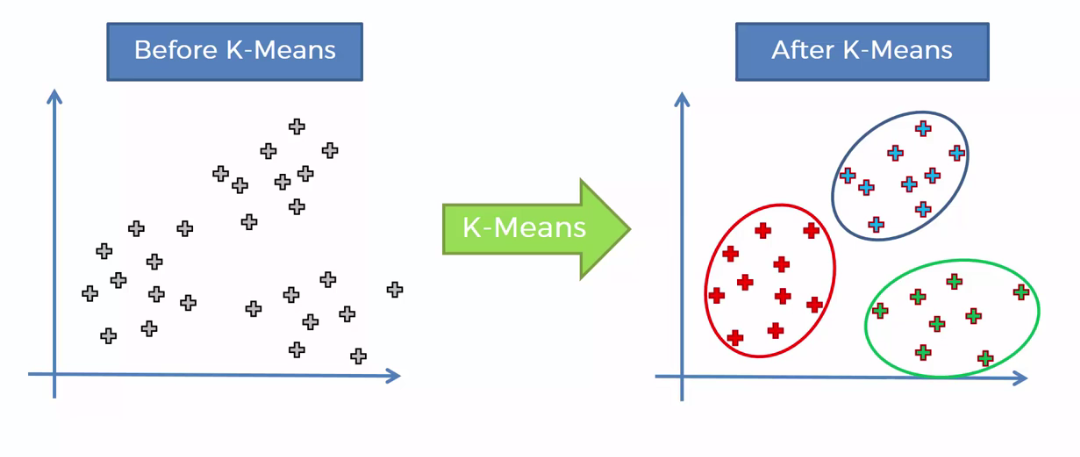K-Means illustration

What are its steps :

1- Initilize K centroids randomly

2- We calculate the distance of each data point from the centroids’ points

3- Assign each point to the closest centroid (using the distance metric)

• Centroid chosen randomly => error : sum of squared distances between points and centroid
• To have less error:

4- Assign new centroids to each cluster (mean for data points in each cluster)

5- Repeat until there are no more changes

In order to Calculate the accuracy, we use two approaches :

• External approach : Compare the clusters with the ground truth, if it’s available
• Internal approach : Average the distance between data points within a cluster

What’s the problem when choosing k ?

Essentially, determining the number of clusters in a data set, or k, as in the k-Means algorithm, is a frequent problem in data clustering. The correct choice of k is often ambiguous, because it’s very dependent on the shape and scale of the distribution of points in a data set. There are some approaches to address this problem, but one of the techniques that is commonly used, is to run the clustering across the different values of K, and looking at a metric of accuracy for clustering. This metric can be “mean distance between data points and their cluster centroid” which indicate how dense our clusters are, or to what extend we minimized the error of clustering. Then looking at the change of this metric, we can find the best value for k. But the problem is that with increasing the number of clusters, the distance of centroids to data points will always reduce. This means, increasing K will always decrease the “error.” So, the value of the metric as a function of K is plotted and the “elbow point” is determined, where the rate of decrease sharply shifts. It is the right K for clustering. This method is called the “elbow” method.

___________________________________________________________________

### — — Agglomerative Hierarchical Clustering (AHC) —

Hierarchical clustering algorithms build a hierarchy of clusters where each node is a cluster consisting of the clusters of its daughter nodes.

What are its steps ?

1- Create n clusters, one for each data point

2- Compute the proximity matrix

3- Repeat :

• merge the two closest clusters
• Update the proximity matrix

4- Until only a single cluster remains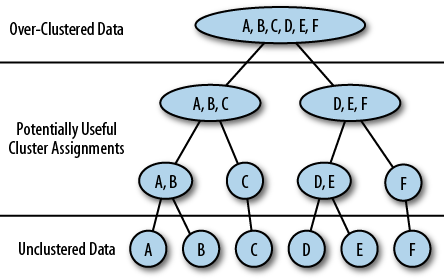AHC illustration

What are the distances used to choose the clusters to merge them ?

• Single-linkage clustering (minimum distance between clusters)
• Complete-linkage clustering (maximum distance between clusters)
• Average linkage clustering (average distance between clusters)
• Centroid linkage clustering (distance between clusters centroids)

Advantages:

• It produces a dendrogram which help understand more the data
• It’s easy to implement

Disadvantages:

• It generally has long runtimes
• It’s sometimes difficult to identify the number of clusters by the dendrogram

___________________________________________________________________

### — — — DBSCAN ( Density-based clustering) — — — —

Why we use it and the difference between K-Means and density-based clustering in terms of anomaly detection:

While partitioning-based algorithms, such as K-Means, may be easy to understand and implement in practice, the algorithm has no notion of outliers. That is, all points are assigned to a cluster, even if they do not belong in any. In the domain of anomaly detection, this causes problems as anomalous points will be assigned to the same cluster as “normal” data points. The anomalous points pull the cluster centroid towards them, making it harder to classify them as anomalous points. In contrast, Density-based clustering locates regions of high density that are separated from one another by regions of low density. Density, in this context, is defined as the number of points within a specified radius. A specific and very popular type of density-based clustering is DBSCAN. DBSCAN is particularly effective for tasks like class identification on a spatial context. The wonderful attribute of the DBSCAN algorithm is that it can find out any arbitrary shape cluster without getting affected by noise.

It’s used to find arbitrary clusters (clusters within clusters)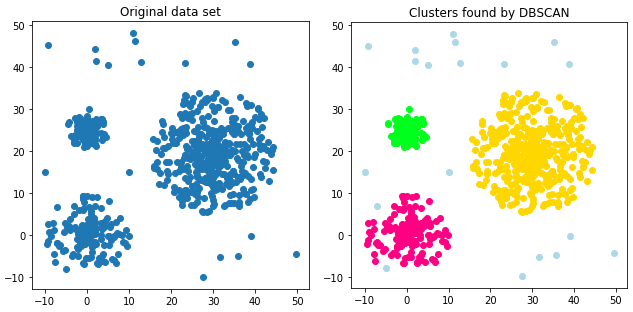DBSCAN illustration

What is it and how it works ?

DBSCAN stands for Density-Based Spatial Clustering of Applications with Noise. This technique is one of the most common clustering algorithms, which works based on density of object.

DBSCAN works on the idea is that if a particular point belongs to a cluster, it should be near to lots of other points in that cluster.

It works based on 2 parameters:

• Radius and Minimum Points. R determines a specified radius that, if it includes enough points within it, we call it a “dense area.”
• M determines the minimum number of data points we want in a neighborhood to define a cluster.

what is a core point? A data point is a core point if, within R-neighborhood of the point, there are at least M points. For example, as there are 6 points in the 2-centimeter neighbor of the red point, we mark this point as a core point.

What is a border point? A data point is a BORDER point if:

a. Its neighbourhood contains less than M data points

OR

b. It is reachable from some core point.

What is an outlier? An outlier is a point that: Is not a core point, and also, is not close enough to be reachable from a core point.

The final step is to connect core points that are neighbors, and put them in the same cluster. So, a cluster is formed as at least one core point, plus all reachable core points, plus all their borders. It simply shapes all the clusters and finds outliers as well.

___________________________________________________________________

___________________________________________________________________

### — — — — — — The Supervised Learning — — — — — —

“Supervised learning is where you have input variables (x) and an output variable (Y) and you use an algorithm to learn the mapping function from the input to the output.
Y = f(X)
The goal is to approximate the mapping function so well that when you have new input data (x) that you can predict the output variables (Y) for that data.
It is called supervised learning because the process of an algorithm learning from the training dataset can be thought of as a teacher supervising the learning process. We know the correct answers, the algorithm iteratively makes predictions on the training data and is corrected by the teacher. Learning stops when the algorithm achieves an acceptable level of performance.” — Machine Learning Mastery

There is two types of unsupervised learning techniques :

• Regression
• Classification

___________________________________________________________________

### Regression

Used mainly to predict a value in the future, regression analysis is a set of statistical processes for estimating the relationships among variables. It includes many techniques for modeling and analyzing several variables, when the focus is on the relationship between a dependent variable Y and one or more independent variables (or ‘predictors’) X . More specifically, regression analysis helps one understand how the typical value of the dependent variable (or ‘criterion variable’) changes when any one of the independent variables is varied, while the other independent variables are held fixed.

There exists two main types of regression :

• Linear regression
• Logistic regression (considered as a classification algorithm)

The difference between both regressions is that Logistic regression is used when the dependent variable is binary in nature. In contrast, Linear regression is used when the dependent variable is continuous and nature of the regression line is linear.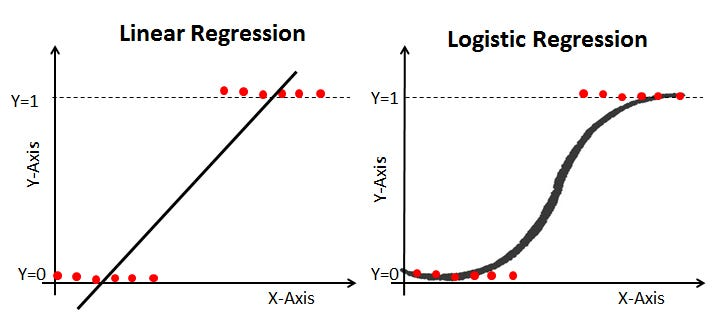Comparison between linear and logistic regression

___________________________________________________________________

### Linear regression

The linear regression technique involves the continuous dependent variable and the independent variables can be continuous or discrete. By using best fit straight line linear regression sets up a relationship between dependent variable (Y) and one or more independent variables (X). In other words, there exist a linear relationship between independent and dependent variables.

A linear regression line has an equation of the form Y = a + bX, where X is the explanatory (independant) variable and Y is the dependent variable.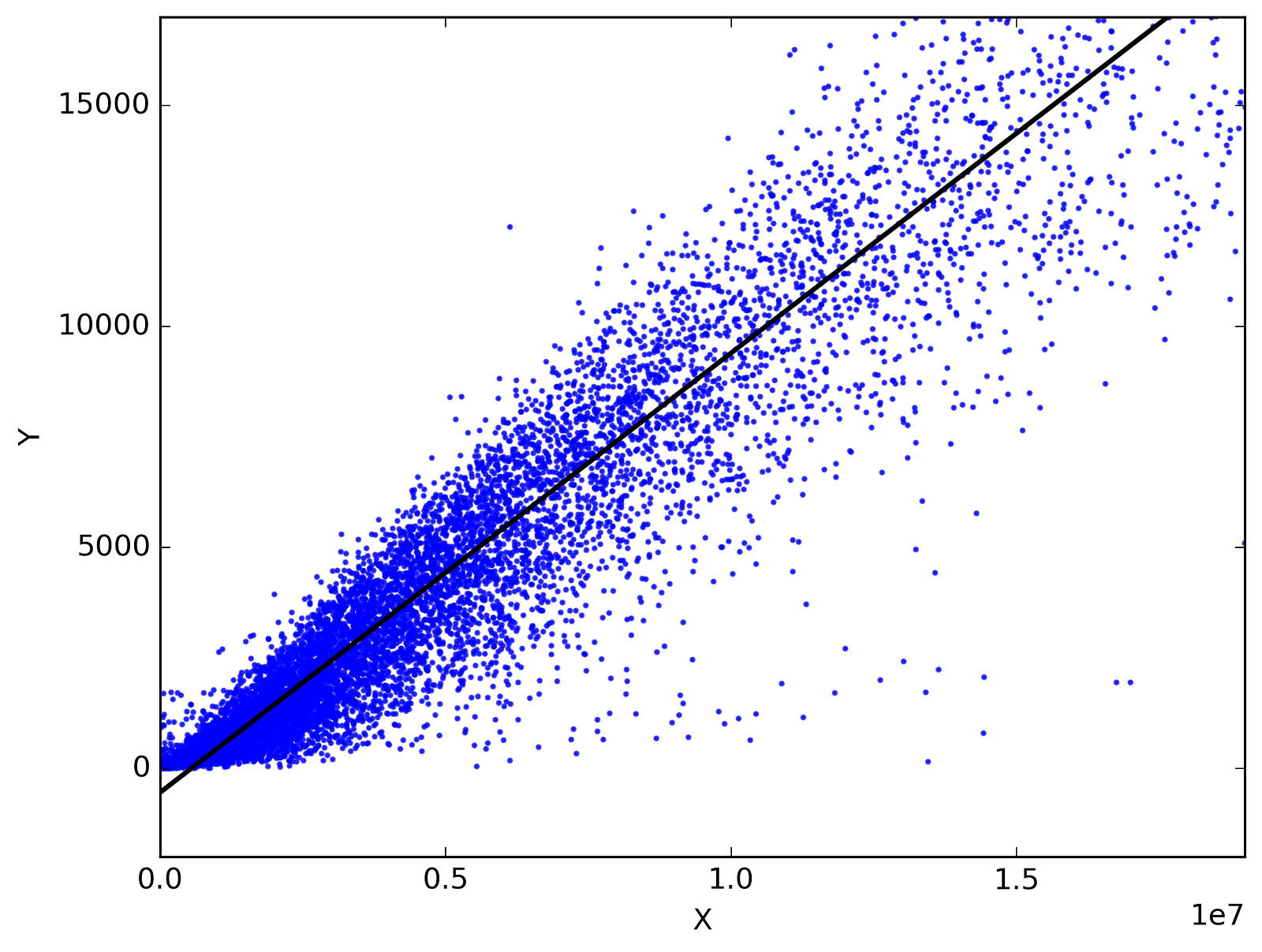Linear Regression illustration

There is two type of linear regression:

• Simple linear regression: one independent variable and the dependant variable
• Multiple linear regression: many independent variables and the dependant variable

How can we evaluate a model ?

There are 3 types of model evaluation/calculating the accuracy :

• Train and test on the same dataset
• Train/Test Split
• K cross-validation

The evaluation metrics are:

• Mean Absolute Error (MAE)
• Mean Squared Error (MSE)
• Root Mean Squared Error (RMSE)
• Relative Absolute Error (RAE)
• Relative Squared Error (RSE)
• R² = 1 — RSE : The higher R² is, the better the model is

An error of the model is the difference between the data points and the trend line generated by the algorithm.

___________________________________________________________________

### — — — — — — — —-Classification — — — — — — — — —

It’s a supervised learning approach whose purpose is to categorize some unknown items into a discrete set of categories or “classes”.

The target attribute is a categorical variable.

Based on the dataset, we do the modelling to have a “Classifier” with which we do the prediction to have the predicted labels

What is it used for?

• Determine to which category a customer belongs
• Determine whether a customer switches to another provider/brand
• Determine whether a customer responds to a particular advertising compaign
• Email filtering
• Speech recognition
• Handwriting recognition
• Biometric identification
• Document classification

What are some of the algorithms used for classification ?

• Decision trees
• Naïve Bayes
• Linear Discriminant Analysis
• K-nearest neighbor
• Logistic regression
• Neural Networks
• Support Vector Machines (SVM)

___________________________________________________________________

### — — — — — — — Logistic regression — — — — — — — — -

What is it used for ?

• Predict the probability of a person having a heart attack
• Predicting the mortality in injured patients
• Predicting a customer’s propensity to purchase a product or halt a subscription
• Predicting the probability of failure of a given process or product
• Predicting the likelihood of a homeowner defaulting on a mortgage

___________________________________________________________________

### — — — — —KNN ( k Nearest Neighbors) — — — — — — -

The k-nearest-neighbors algorithm is a classification algorithm that takes a bunch of labelled points and uses them to learn how to label other points.

This algorithm classifies cases based on their similarity to other cases. In k-nearest neighbors, data points that are near each other are said to be “neighbors.” K-nearest neighbors is based on this paradigm: “Similar cases with the same class labels are near each other.”

Thus, the distance between two cases is a measure of their dissimilarity.

In a classification problem, the k-nearest neighbors algorithm works as follows:

1. Pick a value for K.

2. Calculate the distance from the new case (holdout from each of the cases in the dataset). (Minkawski distance for e.g)

3. Search for the K observations in the training data that are ‘nearest’ to the measurements of the unknown data point.

4. predict the response of the unknown data point using the most popular response value from the K nearest neighbors.

• K is found after trying evaluating, each time, a number of K. We choose the K which lead us to the best accuracy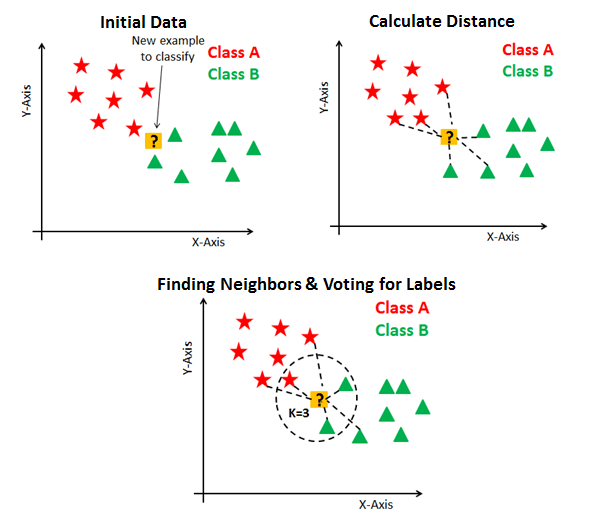KNN illustration

___________________________________________________________________

### — — — — — — —- DECISION TREES — — — — — — — —

Decision Tree learning algorithm generates decision trees from the training data to solve classification and regression problem.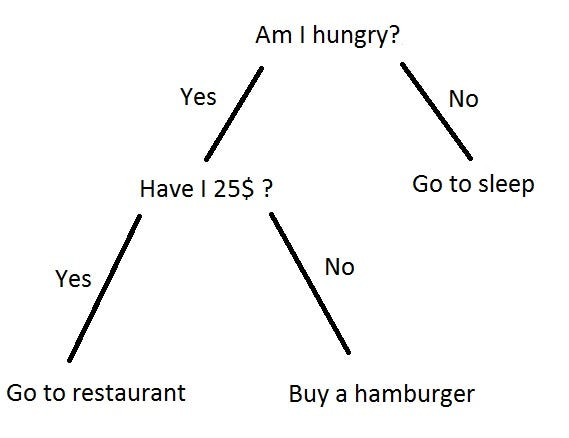Decision Tree illustration

1- Choose an attribute from your dataset

2- Calculate the significance of attribute (indice de gini) in splitting of data

3- Split data based on the value of the best attribute

4- Go to step 1

Entropy is the amount of information disorder, or the amount of randomness in the data. The entropy in the node depends on how much random data is in that node and is calculated for each node. In decision trees, we’re looking for trees that have the smallest entropy in their nodes.

the information gain is the entropy of the tree before the split, minus the weighted entropy after the split.

___________________________________________________________________

### SVM

“Support Vector Machine” (SVM) is a supervised machine learning algorithm which can be used for both classification or regression challenges. However, it is mostly used in classification problems. In this algorithm, we plot each data item as a point in n-dimensional space (where n is number of features you have) with the value of each feature being the value of a particular coordinate. Then, we perform classification by finding the hyper-plane that differentiate the two classes very well (look at the below snapshot).

How does it work ?

1. Mapping data to a high-dimensional feature space

2. Finding a separator

• It’s good for high-dimensional datasets but not small ones
• Use : image recognition, text category assignment, detecting spam, sentiment analysis , gene expression classification, regression, outlier detection and clustering